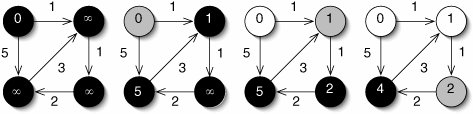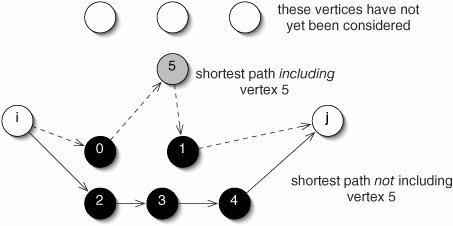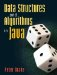# Shortest Paths

A very common graph problem is finding the shortest path between two vertices. Applications range from finding a way through a maze to finding a route through a computer network.

We address the problem for weighted graphs, since the unweighted version is just a special case of this. For example, suppose we want to drive from one city to another. We want a path with the fewest total miles of driving, not the fewest intermediate cities visited. In graph terms, we want to minimize the sum of the weights of the edges on the path, not the number of vertices along the path. This is the shortest-path problem.

It is convenient to talk about the cost to get directly from one vertex to another. This is zero if the vertices are the same one. If the vertices are connected by an edge, the weight of that edge is the cost. If the vertices are not connected by an edge (there is no way to go directly between them), the cost is infinite.

Costs are computed by the method getCost() in Figure 15-30. It makes use of the special double value Double.POSITIVE_INFINITY. This behaves as we would expect infinity to behaveit is greater than any other double, and:

```Double.POSITIVE_INFINITY + 1 == Double.POSITIVE_INFINITY
```

Figure 15-30. The getCost() method returns 0 if i and j are identical, infinity if there is no edge between them, or the weight of the edge if there is one.

 ``` 1 /** Return the cost to go directly from i to j. */ 2 public double getCost(int i, int j) { 3 if (i == j) { 4 return 0.0; 5 } 6 if (edges[i][j] == 0.0) { 7 return Double.POSITIVE_INFINITY; 8 } 9 return edges[i][j]; 10 } ```

We now present two algorithms for finding shortest paths in a weighted graph. For simplicity, we will find the distances rather than the paths themselves. Both algorithms use dynamic programming (Section 9.5). Dijkstra's single-source algorithm determines the distances from one vertex to all others. (We would have to do just as much work to find the distance to a single destination.) The FloydWarshall all-pairs algorithm, which might be used to create a table of distances in a road atlas, determines the distance from each vertex to each other vertex.

Dijkstra's Single-Source Algorithm

Dijkstra's single-source algorithm finds the shortest path from one source vertex to each other vertex. It does this by maintaining an array result containing the distance to each vertex. Initially, the distance to the source vertex is 0 and all other distances are infinite (Figure 15-31).

Figure 15-31. Dijkstra's algorithm at work. Black nodes have not yet been visited. Gray nodes have just been visited. The number within each vertex is its estimated distance. Initially (left) all distances are infinity, except for the distance to the source vertex, which is 0. As each vertex is visited, the distances to its neighbors are updated.As the algorithm runs, it reduces the other distances. By the time each vertex is visited, its distance is correct.

The vertices are visited in order of increasing distance from the source. This guarantees that, by the time we visit a vertex, we have visited all of the vertices along the shortest path to that vertex. This is similar to a breadth-first traversal, but the weights are taken into account. As each vertex i is visited, the distances to its neighbors are updated. Specifically, we compare the current distance of neighbor j (that is, result[j]) with the length of the path going from the source to i, then following one edge from i to j:

```result[i] + getCost(i, j)
```

If this sum is smaller, we have found a shorter path to j, so we update result[j].

The code for this algorithm is given in Figure 15-32. The main loop on lines 2229 runs v times. On each pass, it invokes cheapest() and runs the inner loop on lines 2528, each of which take time in Q(v). The total running time is therefore in Q(v2).

Figure 15-32. Code for Dijkstra's algorithm.

 ``` 1 /** 2 * Return the index of the smallest element of distances, 3 * ignoring those in visited. 4 */ 5 protected int cheapest(double[] distances, boolean[] visited) { 6 int best = -1; 7 for (int i = 0; i < size(); i++) { 8 if (!visited[i] 9 && ((best < 0) || (distances[i] < distances[best]))) { 10 best = i; 11 } 12 } 13 return best; 14 } 15 ``` 16 /** 17 /* Return an array of the distances from source to each other 18 * vertex. 19 */ 20 public double[] distancesFrom(int source) { 21 double[] result = new double[size()]; 22 java.util.Arrays.fill(result, Double.POSITIVE_INFINITY); 23 result[source] = 0; 24 boolean[] visited = new boolean[size()]; 25 for (int i = 0; i < size(); i++) { 26 int vertex = cheapest(result, visited); 27 visited[vertex] = true; 28 for (int j = 0; j < size(); j++) { 29 result[j] = Math.min(result[j], 30 result[vertex] + getCost(vertex, j)); 31 } 32 } 33 return result; 34 }

The FloydWarshall All-Pairs Algorithm

To find the shortest path between each pair of vertices, we could just run Dijkstra's algorithm once from each vertex, using time in Q(v3). The FloydWarshall all-pairs algorithm takes time in this order, but it is somewhat simpler, so there is a smaller constant factor associated with the asymptotic notation. It is also somewhat easier to write.

Like Dijkstra's algorithm, the FloydWarshall all-pairs algorithm uses dynamic programming. Specifically, it maintains a two-dimensional result array, where result[i][j] is the shortest known distance from vertex i to vertex j.

Initially, all of the elements are initialized to the costs, as computed by getCost(). The FloydWarshall algorithm updates the result by asking the following series of questions:

• What is the shortest distance between each pair of vertices using vertex 0 as an intermediate point?
• What is the shortest distance between each pair of vertices using vertices 0 and 1 as intermediate points?
• What is the shortest distance between each pair of vertices using vertices 0 through 2 as intermediate points?

This continues until all possible intermediate points have been considered, at which point the distances are correct.

To see how the updating works, suppose we have considered vertices 0 through 4 as intermediate points, and we're ready to consider 0 through 5 (Figure 15-33). There are two possibilities for each pair of vertices i and j:

• The shortest path using vertices 0 through 5 as intermediate points does not involve vertex 5. It was already correct.
• The shortest path using vertices 0 through 5 as intermediate points does involve vertex 5. It must be result[i] + result[j]. Neither of these two subpaths can have vertex 5 as an intermediate point, because it is an endpoint.

Figure 15-33. Finding the shortest path from vertex i to vertex j, using only vertices 0 through 5 as intermediate points. The shortest distance must be either result[i][j] (lower path) or result[i] + result[j] (upper path). This diagram is schematic; many other edges, not shown, are presumed to exist.Updating result consists of taking the minimum of these two possibilities for each pair of vertices.

The code (Figure 15-34) is short, sweet, and to the point. Lines 1119 are a simple triply nested loop, giving a running time in Q(v3).

Figure 15-34. The FloydWarshall all-pairs algorithm.

 ```1 /** 2 * Return a two-dimensional array of the distances from each vertex 3 * to each other vertex. 4 */ 5 public double[][] distances() { 6 double[][] result = new double[size()][size()]; ``` 7 for (int i = 0; i < size(); i++) { 8 for (int j = 0; j < size(); j++) { 9 result[i][j] = getCost(i, j); 10 } 11 } 12 for (int midpoint = 0; midpoint < size(); midpoint++) { 13 for (int i = 0; i < size(); i++) { 14 for (int j = 0; j < size(); j++) { 15 result[i][j] = Math.min(result[i][j], 16 result[i][midpoint] 17 + result[midpoint][j]); 18 } 19 } 20 } 21 return result; 22 }

Exercises

 15.19 What is the value of 1 / 0? What about 1.0 / 0? 15.2 Write a Graph method which takes two vertex numbers and returns the distance between them. 15.21 What is the order of the running time of the getCost() method (Figure 15-30)? What would it be if Graph used the neighbor list representation instead of the neighbor matrix representation? 15.22 Would the algorithms in this section work on unweighted graphs? Explain.

### Minimum Spanning TreesData Structures and Algorithms in Java
ISBN: 0131469142
EAN: 2147483647
Year: 2004
Pages: 216
Authors: Peter Drake